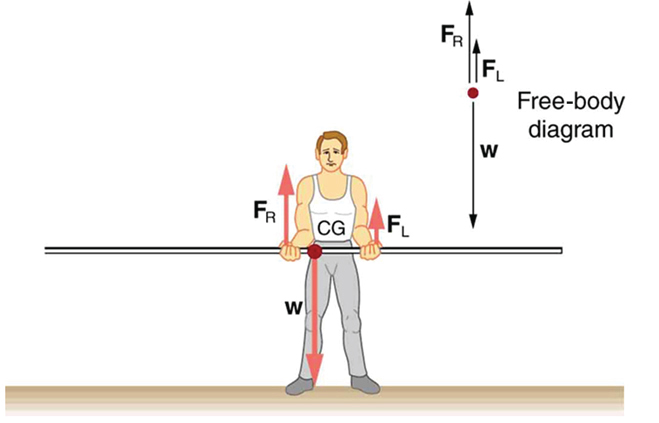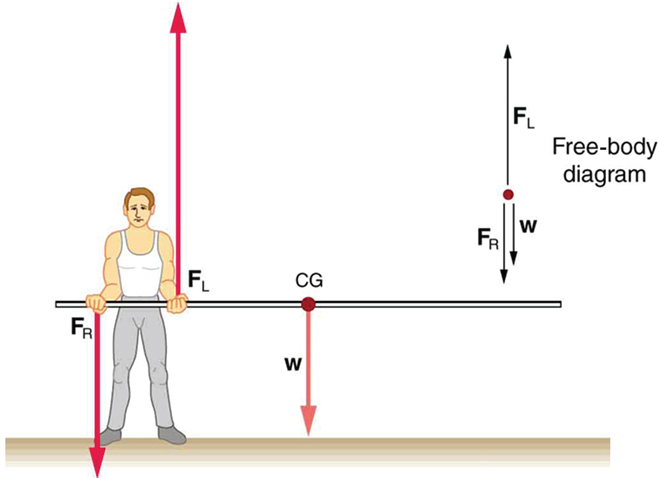# 9.4 Applications of statics, including problem-solving strategies  (Page 2/3)

 Page 2 / 3

Similar observations can be made using a meter stick held at different locations along its length.A pole vaulter is holding a pole horizontally with both hands. The center of gravity is near his right hand.A pole vaulter is holding a pole horizontally with both hands. The center of gravity is to the left side of the vaulter.

If the pole vaulter holds the pole as shown in [link] , the situation is not as simple. The total force he exerts is still equal to the weight of the pole, but it is not evenly divided between his hands. (If ${F}_{L}={F}_{R}$ , then the torques about the cg would not be equal since the lever arms are different.) Logically, the right hand should support more weight, since it is closer to the cg. In fact, if the right hand is moved directly under the cg, it will support all the weight. This situation is exactly analogous to two people carrying a load; the one closer to the cg carries more of its weight. Finding the forces ${F}_{L}$ and ${F}_{R}$ is straightforward, as the next example shows.

If the pole vaulter holds the pole from near the end of the pole ( [link] ), the direction of the force applied by the right hand of the vaulter reverses its direction.

## What force is needed to support a weight held near its cg?

For the situation shown in [link] , calculate: (a) ${F}_{R}$ , the force exerted by the right hand, and (b) ${F}_{L}$ , the force exerted by the left hand. The hands are 0.900 m apart, and the cg of the pole is 0.600 m from the left hand.

Strategy

[link] includes a free body diagram for the pole, the system of interest. There is not enough information to use the first condition for equilibrium $\text{(net}\phantom{\rule{0.25em}{0ex}}F=0$ ), since two of the three forces are unknown and the hand forces cannot be assumed to be equal in this case. There is enough information to use the second condition for equilibrium $\text{(net}\phantom{\rule{0.25em}{0ex}}\tau =0\text{)}$ if the pivot point is chosen to be at either hand, thereby making the torque from that hand zero. We choose to locate the pivot at the left hand in this part of the problem, to eliminate the torque from the left hand.

Solution for (a)

There are now only two nonzero torques, those from the gravitational force ( ${\tau }_{\text{w}}$ ) and from the push or pull of the right hand ( ${\tau }_{R}$ ). Stating the second condition in terms of clockwise and counterclockwise torques,

$\text{net}\phantom{\rule{0.25em}{0ex}}{\tau }_{\text{cw}}=\text{–net}\phantom{\rule{0.25em}{0ex}}{\tau }_{\text{ccw}}\text{.}$

or the algebraic sum of the torques is zero.

Here this is

${\tau }_{R}={\mathrm{–\tau }}_{\text{w}}$

since the weight of the pole creates a counterclockwise torque and the right hand counters with a clockwise torque. Using the definition of torque, $\tau =\text{rF}\phantom{\rule{0.25em}{0ex}}\text{sin}\phantom{\rule{0.25em}{0ex}}\theta$ , noting that $\theta =90º$ , and substituting known values, we obtain

$\left(0\text{.}\text{900 m}\right)\left({F}_{R}\right)=\left(0\text{.600 m}\right)\left(\mathit{mg}\right)\text{.}$

Thus,

$\begin{array}{lll}{F}_{R}& =& \left(0.667\right)\left(\text{5.00 kg}\right)\left(9.80\phantom{\rule{0.25em}{0ex}}{\text{m/s}}^{2}\right)\\ & =& \text{32.7 N.}\end{array}$

Solution for (b)

The first condition for equilibrium is based on the free body diagram in the figure. This implies that by Newton’s second law:

${F}_{L}+{F}_{R}–\text{mg}=0$

From this we can conclude:

${F}_{L}+{F}_{R}=w=\text{mg}$

Solving for ${F}_{L}$ , we obtain

$\begin{array}{lll}{F}_{L}& =& \mathit{mg}-{F}_{R}\\ & =& \mathit{mg}-\text{32}\text{.}7 N\\ & =& \left(\text{5.00 kg}\right)\left(\text{9.80}\phantom{\rule{0.25em}{0ex}}{\text{m/s}}^{2}\right)-\text{32.7 N}\\ & =& \text{16.3 N}\end{array}$

Discussion

${F}_{L}$ is seen to be exactly half of ${F}_{R}$ , as we might have guessed, since ${F}_{L}$ is applied twice as far from the cg as ${F}_{R}$ .

If the pole vaulter holds the pole as he might at the start of a run, shown in [link] , the forces change again. Both are considerably greater, and one force reverses direction.

## Take-home experiment

This is an experiment to perform while standing in a bus or a train. Stand facing sideways. How do you move your body to readjust the distribution of your mass as the bus accelerates and decelerates? Now stand facing forward. How do you move your body to readjust the distribution of your mass as the bus accelerates and decelerates? Why is it easier and safer to stand facing sideways rather than forward? Note: For your safety (and those around you), make sure you are holding onto something while you carry out this activity!

## Phet explorations: balancing act

Play with objects on a teeter totter to learn about balance. Test what you've learned by trying the Balance Challenge game.

## Summary

• Statics can be applied to a variety of situations, ranging from raising a drawbridge to bad posture and back strain. We have discussed the problem-solving strategies specifically useful for statics. Statics is a special case of Newton’s laws, both the general problem-solving strategies and the special strategies for Newton’s laws, discussed in Problem-Solving Strategies , still apply.

## Conceptual questions

When visiting some countries, you may see a person balancing a load on the head. Explain why the center of mass of the load needs to be directly above the person’s neck vertebrae.

## Problems&Exercises

To get up on the roof, a person (mass 70.0 kg) places a 6.00-m aluminum ladder (mass 10.0 kg) against the house on a concrete pad with the base of the ladder 2.00 m from the house. The ladder rests against a plastic rain gutter, which we can assume to be frictionless. The center of mass of the ladder is 2 m from the bottom. The person is standing 3 m from the bottom. What are the magnitudes of the forces on the ladder at the top and bottom?

In [link] , the cg of the pole held by the pole vaulter is 2.00 m from the left hand, and the hands are 0.700 m apart. Calculate the force exerted by (a) his right hand and (b) his left hand. (c) If each hand supports half the weight of the pole in [link] , show that the second condition for equilibrium $\text{(net}\phantom{\rule{0.25em}{0ex}}{\tau }_{}=\text{0)}$ is satisfied for a pivot other than the one located at the center of gravity of the pole. Explicitly show how you follow the steps in the Problem-Solving Strategy for static equilibrium described above.

write an expression for a plane progressive wave moving from left to right along x axis and having amplitude 0.02m, frequency of 650Hz and speed if 680ms-¹
how does a model differ from a theory
what is vector quantity
Vector quality have both direction and magnitude, such as Force, displacement, acceleration and etc.
Besmellah
Is the force attractive or repulsive between the hot and neutral lines hung from power poles? Why?
what's electromagnetic induction
electromagnetic induction is a process in which conductor is put in a particular position and magnetic field keeps varying.
Lukman
wow great
Salaudeen
what is mutual induction?
je
mutual induction can be define as the current flowing in one coil that induces a voltage in an adjacent coil.
Johnson
how to undergo polarization
show that a particle moving under the influence of an attractive force mu/y³ towards the axis x. show that if it be projected from the point (0,k) with the component velocities U and V parallel to the axis of x and y, it will not strike the axis of x unless u>v²k² and distance uk²/√u-vk as origin
show that a particle moving under the influence of an attractive force mu/y^3 towards the axis x. show that if it be projected from the point (0,k) with the component velocities U and V parallel to the axis of x and y, it will not strike the axis of x unless u>v^2k^2 and distance uk^2/√u-k as origin
No idea.... Are you even sure this question exist?
Mavis
I can't even understand the question
yes it was an assignment question "^"represent raise to power pls
Gabriel
Gabriel
An engineer builds two simple pendula. Both are suspended from small wires secured to the ceiling of a room. Each pendulum hovers 2 cm above the floor. Pendulum 1 has a bob with a mass of 10kg . Pendulum 2 has a bob with a mass of 100 kg . Describe how the motion of the pendula will differ if the bobs are both displaced by 12º .
no ideas
Augstine
if u at an angle of 12 degrees their period will be same so as their velocity, that means they both move simultaneously since both both hovers at same length meaning they have the same length
Modern cars are made of materials that make them collapsible upon collision. Explain using physics concept (Force and impulse), how these car designs help with the safety of passengers.
calculate the force due to surface tension required to support a column liquid in a capillary tube 5mm. If the capillary tube is dipped into a beaker of water
find the time required for a train Half a Kilometre long to cross a bridge almost kilometre long racing at 100km/h
method of polarization
Ajayi
What is atomic number?
The number of protons in the nucleus of an atom
Deborah
type of thermodynamics
oxygen gas contained in a ccylinder of volume has a temp of 300k and pressure 2.5×10Nm
why the satellite does not drop to the earth explain
what is a matter
Yinka
what is matter
Yinka
what is matter
Yinka
what is a matter
Yinka
I want the nuclear physics conversation
Mohamed
because space is a vacuum and anything outside the earth 🌎 can not come back without an act of force applied to it to leave the vacuum and fall down to the earth with a maximum force length of 30kcm per second
Clara

#### Get Jobilize Job Search Mobile App in your pocket Now!ByByBy Richley CrapoBy Brooke DelaneyBy Madison ChristianBy Sandhills MLTBy OpenStaxBy Kevin MoquinBy OpenStaxBy Sean WiffleBoyBy Abby SharpBy Heather McAvoy